Algebra 1 1-9 Complete Lesson: Patterns, Equations, and Graphs
starstarstarstarstarstarstarstarstarstar
5 (1 rating)
by Matthew Richardson
| 32 Questions
Note from the author:
A complete formative lesson with embedded slideshow, mini lecture screencasts, checks for understanding, practice items, mixed review, and reflection. I create these assignments to supplement each lesson of Pearson's Common Core Edition Algebra 1, Algebra 2, and Geometry courses. See also mathquest.net and twitter.com/mathquestEDU.The outlined content above was added from outside of Formative.1
2
1
10 pts
Solve It! The table shows the relationship between the number of kites in an arrangement and the total number of ribbons on the kites' tails.

Describe the pattern in the table.
2
10 pts
How many kites could you make with 275 ribbons?

Enter only a number.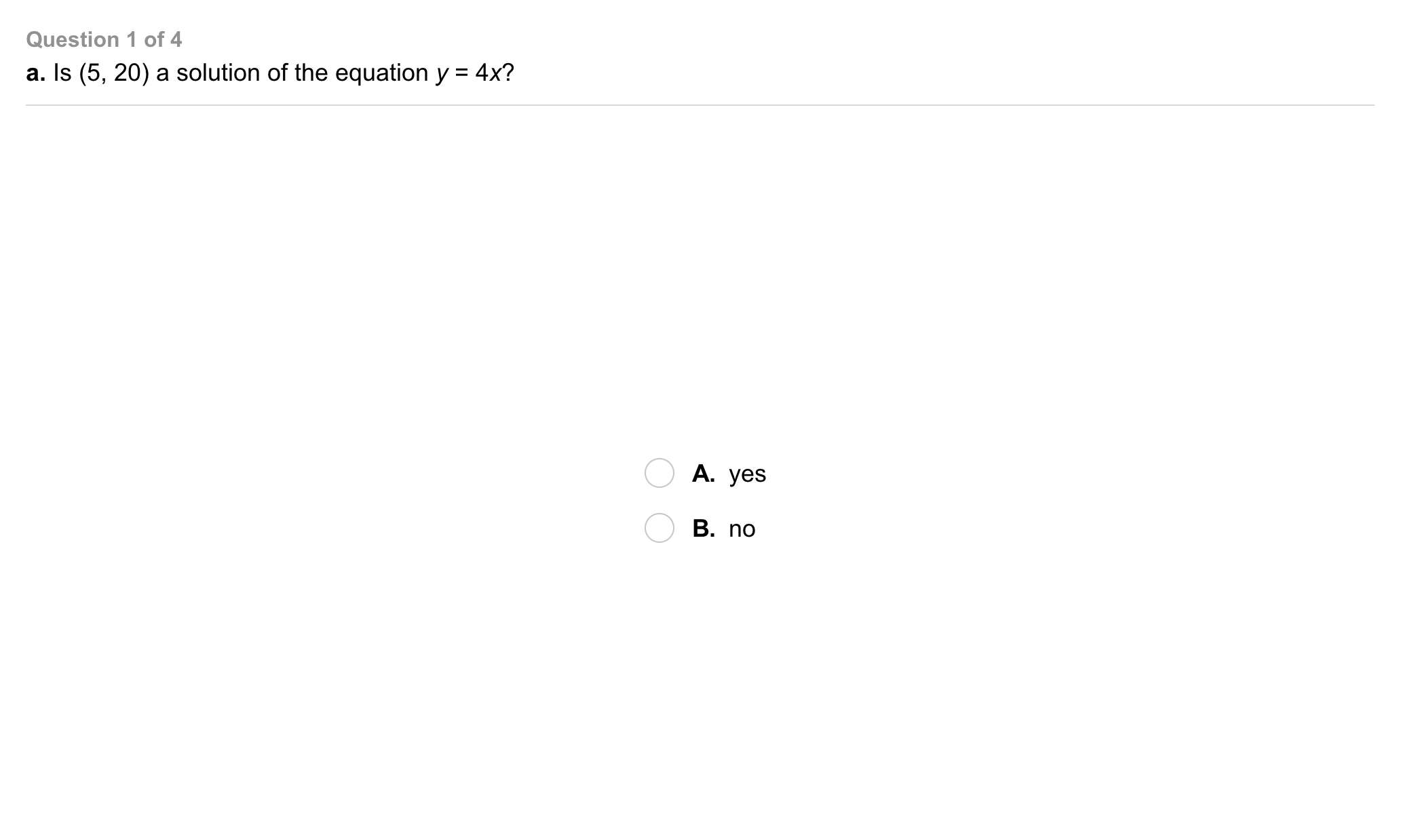3
3
5 pts
Problem 1 Got It?
A
B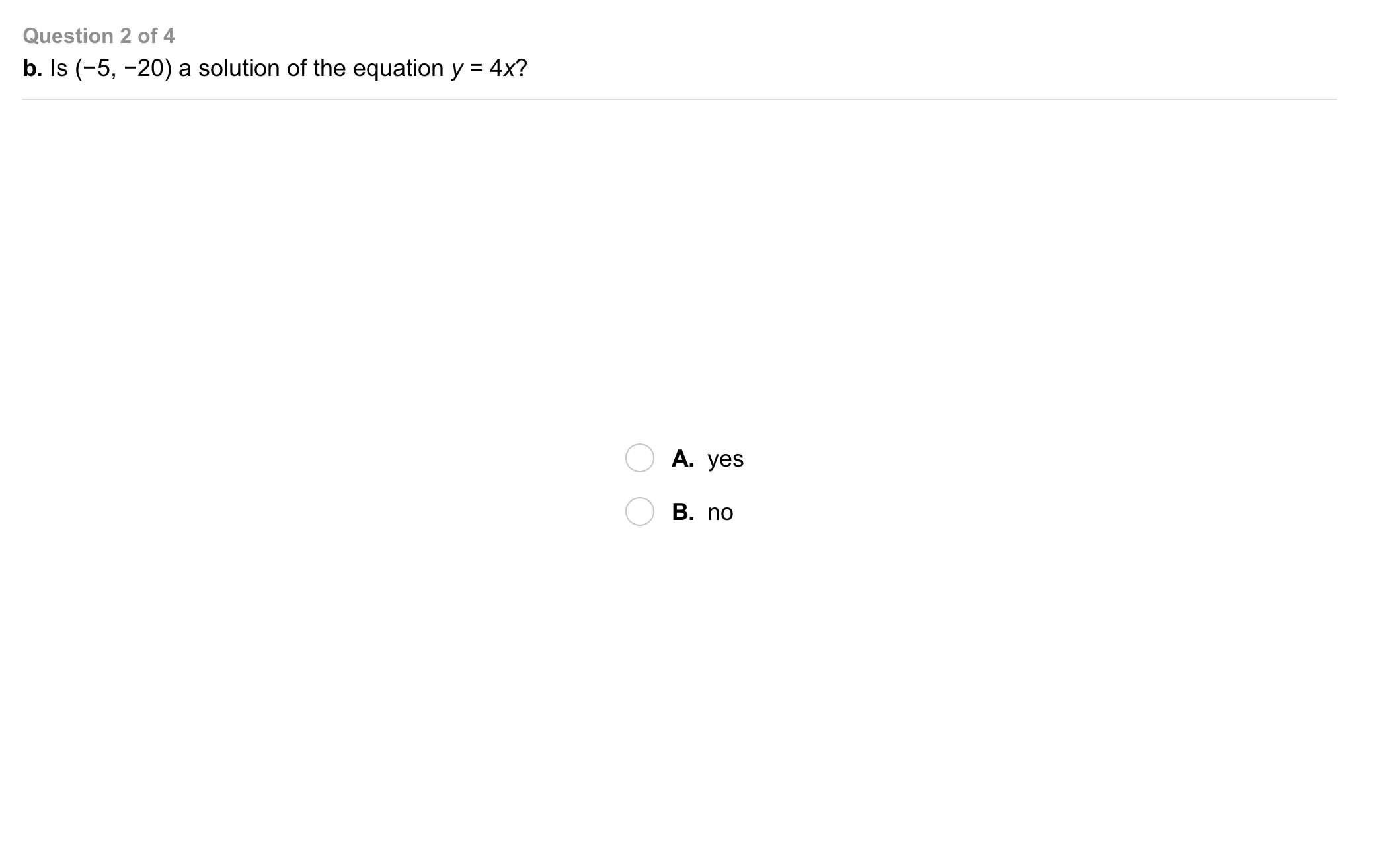4
4
5 pts
Problem 1 Got It?
A
B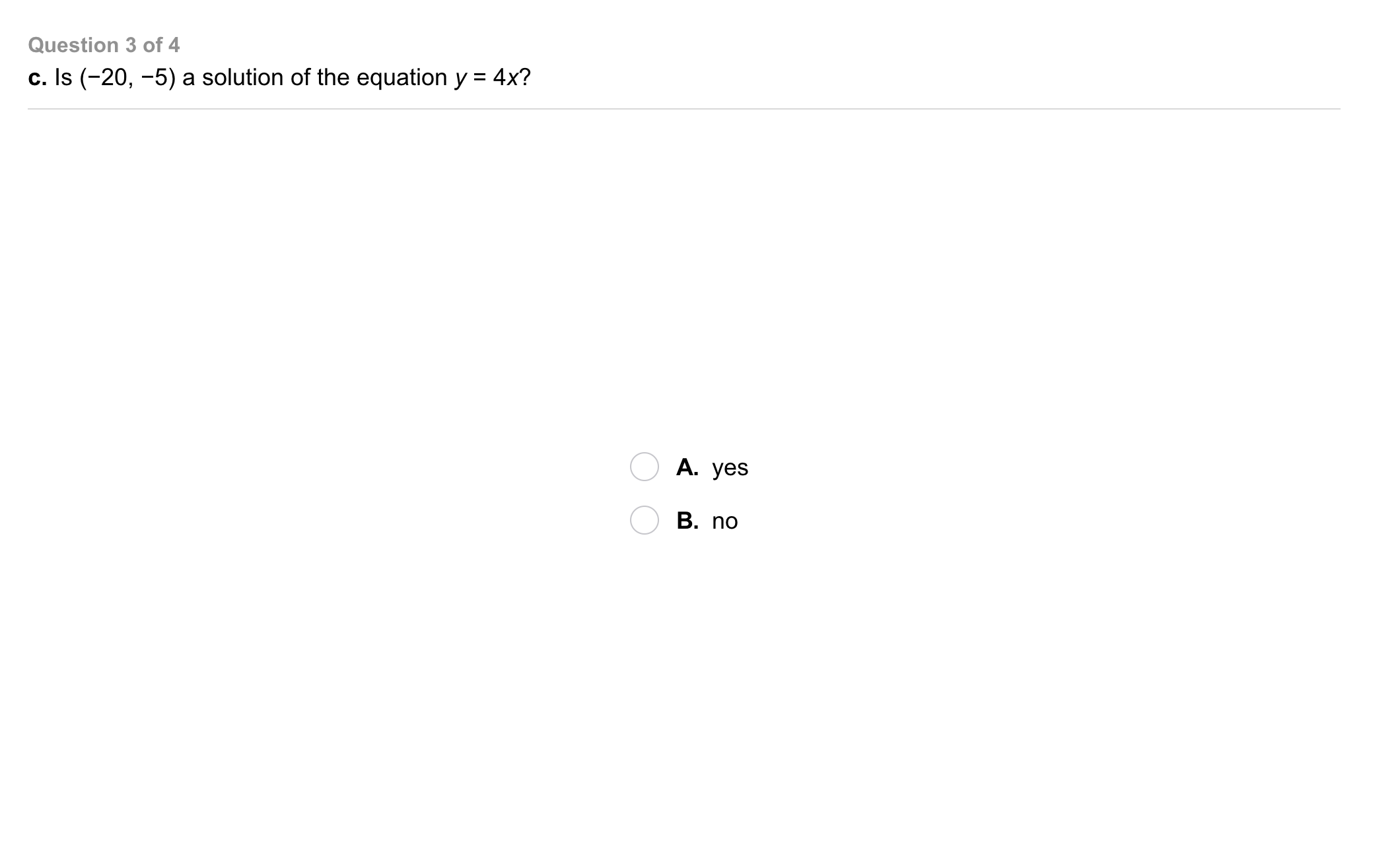5
5
5 pts
Problem 1 Got It?
A
B6
6
5 pts
Problem 1 Got It?
A
B
7
10 pts
Problem 2 Got It? Will runs 6 laps before Megan joins him at the track. They run together at the same pace. How can you represent the relationship between the number of laps Will runs and the number of laps Megan runs in a table?
8
10 pts
Problem 2 Got It? Will runs 6 laps before Megan joins him at the track. They run together at the same pace. How can you represent the relationship between the number of laps Will runs and the number of laps Megan runs on a graph?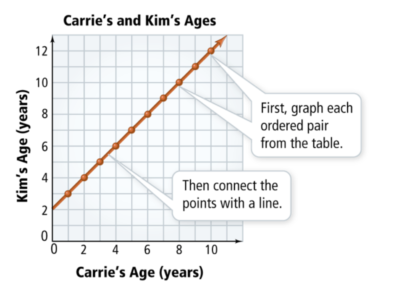9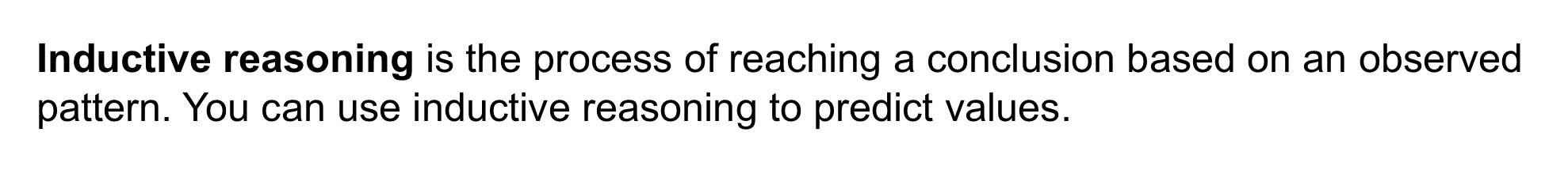10
11
10
10 pts
Problem 3 Got It? Use the figure from problem 3 to make a table showing the number of orange tiles and the total number of tiles in each figure.
11
10 pts
Problem 3 Got It? How many tiles in all will be in a figure with 24 orange tiles?

Enter only a number.12
13
12
10 pts
Problem 3 Got It? Use the figure from problem 3 to make a table showing the number of blue tiles and the number of yellow tiles in each figure.
13
10 pts
Problem 3 Got It? How many yellow tiles will be in a figure with 24 blue tiles?

Enter only a number.14
5 pts
Is (2, 4) a solution of the equation?

Yes
No
15
5 pts
Is (-3, -9) a solution of the equation?

No
Yes
16
60 pts
Drinks at the fair cost \$2.50, Use a table, an equation, and a graph to represent the relationship between the number of drinks bought and the cost.
17
10 pts
Exercise: On a treadmill, you burn 11 Cal in 1 min, 22 Cal in 2 min, 33 Cal in 3 minutes and so on. How many Calories do you burn in 10 min?
Enter only the number of calories.19
10 pts
Reasoning: Which of (3, 5), (4, 6), (5, 7), and (6, 8) are solutions of y = x + 2? What is the pattern in the solutions of y = x + 2?
• (3, 5)
• (4, 6)
• (5, 7)
• (6, 8)
• The y-value is always twice the x-value.
• The y-value is always two more than the x-value.
• Solution(s) of y = x + 2
• The pattern in the solutions of y = x + 220
5 pts
Review Lesson 1-8: Tell whether the given number is a solution of the equation.

yes
no
21
5 pts
Review Lesson 1-8: Tell whether the given number is a solution of the equation.

no
yes
22
5 pts
Review Lesson 1-8: Tell whether the given number is a solution of the equation.

yes
no
23
10 pts
Review Lesson 1-4: Give an example that illustrates the Comutative Property of Addition.
2 ⋅ 1 = 2
-23 = 0 + (-23)
7 + 4 = 4 + 7
(6 ⋅ 3) ⋅ x = 6 ⋅ (x ⋅ 3)
24
10 pts
Review Lesson 1-4: Give an example that illustrates the Associative Property of Multiplication.
(6 ⋅ 3) ⋅ y = 6 ⋅ (y ⋅ 3)
7 + 4 = 4 + 7
2 ⋅ 1 = 2
-23 = 0 + (-23)
25
10 pts
Review Lesson 1-4: Give an example that illustrates the Identity Property of Multiplication.
7 + 4 = 4 + 7
-23 = 0 + (-23)
(6 ⋅ 3) ⋅ x = 6 ⋅ (x ⋅ 3)
2 ⋅ 1 = 2
26
10 pts
Review Lesson 1-4: Give an example that illustrates the Zero Property of Addition.
2 ⋅ 1 = 2
(6 ⋅ 3) ⋅ x = 6 ⋅ (x ⋅ 3)
-23 = 0 + (-23)
7 + 4 = 4 + 7
27
10 pts
Review Lesson 1-5: Find the difference.
Enter only a number.
28
10 pts
Review Lesson 1-5: Find the sum.
Enter only a number.29
10 pts
Vocabulary Review: Classify each pair in the left column as opposites (aka additive inverses) or reciprocals (aka multiplicative inverses).

• Opposites
• Reciprocals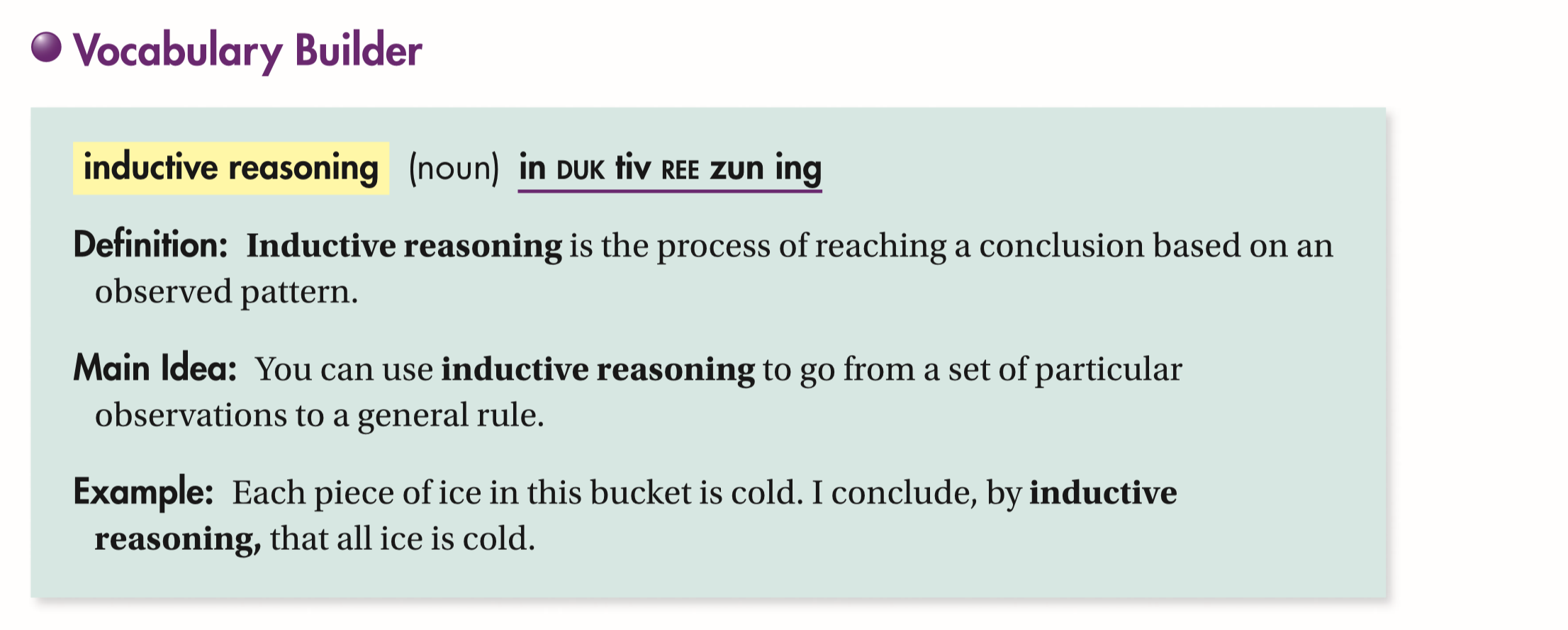30
10 pts
Use Your Vocabulary: Use the table below to complete each statement.

• inductive reasoning
• multiply
• pattern
• subtract
• To find the value of Item 5, you can look for a __?__.
• To obtain the value for an item, you can __?__ the item number by itself.
• You can use __?__ to predict the value of Item 5.
31
100 pts
Notes: Take a clear picture or screenshot of your Cornell notes for this lesson. Upload it to the canvas. Zoom and pan as needed.

For a refresher on the Cornell note-taking system, click here.
32
10 pts
Reflection: Math Success
Add to my formatives list

Formative uses cookies to allow us to better understand how the site is used. By continuing to use this site, you consent to the Terms of Service and Privacy Policy.